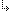- 4.45.2.59. -4. Standard Units4.45. Math - Extended Mathematics unit4.45.2. Math Unit Procedures and Functions4.45.2.59. Sln function

## 4.45.2.59. Sln function

Targets: MS-DOS, OS/2, Win32

Math Unit

Returns the straight-line depreciation allowance of an asset.

Declaration:
```function Sln(InitialValue,
Residue: Extended;
Time: DWord): Extended;```
Remarks:
The Sln function calculates the straight-line depreciation allowance for an asset over one period of its life. The function divides the InitialValue minus the Residue by the number of years of useful Time of the asset. InitialValue is the amount initially paid for the asset. Residue is the value of the asset at the end of its useful life.

- 4.45.2.59. -